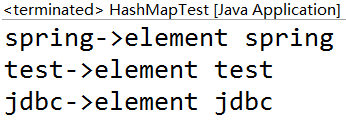jisonami A Java Dev Engineer

# Java集合框架（二）--Java8新增的函数式集合操作方式

### 简单说一说Java8的新特性

#### lambda表达式

lambda表达式：一种将类似数学表达式赋值给变量的语法，这个数学表达式其实就是被语法糖简化了的函数。

lambda有点像Java8之前的匿名内部类，其实不是。它们在JVM中的底层实现方式是不一样的，所以造成的结果也不完全一样。

lambda与匿名内部类一个明显的区别就是this指针的引用。匿名内部类的this是指向该匿名内部类本身，而lambda则是指向使用该lambda表达式的对象。

Java8的lambda表达式是作为实现只有单个抽象方法的接口来使用的。这种接口也叫函数式接口。

#### 重新设计的接口语法

Java8的接口语法被重新设计了，原本接口是全部都是抽象函数的OOP契约，现在接口中可以有很多default默认方法（其实就是实例方法）和static静态方法。

#### 流式编程

Java8的流式编程，这个才是Java8函数编程的核心，通过在集合框架的顶层接口中新增default方法的方式，为原本无法扩展的集合API提供了函数式编程的入口方法，比如stream（）方法和parallelStream()方法。

### Java8新的集合操作方式

#### Iterator接口中新增的foreach()默认方法

``````default void forEach(Consumer<? super T> action) {
Objects.requireNonNull(action);
for (T t : this) {
action.accept(t);
}
}
``````

``````    for (Integer e : ad) {
System.out.println(e);
}
``````

``````ad.forEach(e -> System.out.println(e));
``````

#### Collection接口新增的removeIf()默认方法

``````default boolean removeIf(Predicate<? super E> filter) {
Objects.requireNonNull(filter);
boolean removed = false;
final Iterator<E> each = iterator();
while (each.hasNext()) {
if (filter.test(each.next())) {
each.remove();
removed = true;
}
}
return removed;
}
``````

``````    List<Integer> al = new ArrayList<Integer>();
``````

``````    Iterator<Integer> it = al.iterator();
while(it.hasNext()){
if(it.next()>3){
it.remove();
}
}
``````

``````al.removeIf(e -> e>3);
``````

#### Collection接口新增的stream()和parallelStream()方法

stream()和parallelStream()方法都是获取了一个Stream对象，之后的编程方式是一样。

parallelStream()得到的是并行流，也就是流对象的操作可能是多线程同时进行的，然后将结果合并到一起。

``````    List<Integer> al = new ArrayList<Integer>();
``````

``````    int sum = 0;
for (Integer e : al) {
if(e >4){
sum = sum + e*2;
}
}
``````

``````int sum = al.stream()
.filter(e -> e>4)
.map(e -> e*2)
.reduce((e1,e2) -> (e1+e2)).get();
``````

``````int sum = al.stream()
.filter(e -> e>4)
.map(e -> e*2)
.collect(Collectors.summingInt(e -> e));
``````

#### List接口新增的sort()默认方法

``````default void sort(Comparator<? super E> c) {
Object[] a = this.toArray();
Arrays.sort(a, (Comparator) c);
ListIterator<E> i = this.listIterator();
for (Object e : a) {
i.next();
i.set((E) e);
}
}
``````

``````class KeyTest{
int value;

public KeyTest(int value){
this.value = value;
}

public int getValue() {
return value;
}

}
``````

``````    List<KeyTest> al = new ArrayList< KeyTest>();
``````

``````al.sort((e1,e2) -> Integer.valueOf(e1.getValue()).compareTo(e2.getValue()));
``````

``````class KeyTest implements Comparable<KeyTest>{
int value;

public KeyTest(int value){
this.value = value;
}

public int getValue() {
return value;
}

@Override
public int compareTo(KeyTest o) {
return Integer.valueOf(this.value).compareTo(o.value);
}
}
``````

``````al.sort(null);
``````

#### Map接口新增的forEach()默认方法

``````default void forEach(BiConsumer<? super K, ? super V> action) {
Objects.requireNonNull(action);
for (Map.Entry<K, V> entry : entrySet()) {
K k;
V v;
try {
k = entry.getKey();
v = entry.getValue();
} catch(IllegalStateException ise) {
// this usually means the entry is no longer in the map.
throw new ConcurrentModificationException(ise);
}
action.accept(k, v);
}
}
``````

``````map.forEach((k, v) -> System.out.println(k + "->" + v));
``````

#### Map接口新增的replaceAll()默认方法

``````default void replaceAll(BiFunction<? super K, ? super V, ? extends V> function) {
Objects.requireNonNull(function);
for (Map.Entry<K, V> entry : entrySet()) {
K k;
V v;
try {
k = entry.getKey();
v = entry.getValue();
} catch(IllegalStateException ise) {
// this usually means the entry is no longer in the map.
throw new ConcurrentModificationException(ise);
}

// ise thrown from function is not a cme.
v = function.apply(k, v);

try {
entry.setValue(v);
} catch(IllegalStateException ise) {
// this usually means the entry is no longer in the map.
throw new ConcurrentModificationException(ise);
}
}
}
``````

``````v = function.apply(k, v);
``````

``````    Map<String, String> map = new HashMap<String, String>();
map.put("test", "value test");
map.put("jdbc", "value jdbc");
map.put("spring", "value spring");
``````

``````map.replaceAll((k, v) -> v.replaceAll("value", "element"));
``````#### Map接口其余的默认方

• 若key值对应的value为null，则返回defaultValue
``````V getOrDefault(Object key, V defaultValue)
``````
• 若key值对应为value为null，设值为value，否则不操作
``````V putIfAbsent(K key, V value)
``````
• 若key值对应的value为null，根据mappingFunction计算的值设值，否则不操作
``````V computeIfAbsent(K key, Function<? super K, ? extends V> mappingFunction)
``````
• 若key值对应的value不为空，根据remappingFunction计算的值设值，否则不操作
``````V computeIfPresent(K key, BiFunction<? super K, ? super V, ? extends V> remappingFunction)
``````
• 根据remappingFunction计算的值对key对应的value设值，若计算的值为null，则删除key-value键值对
``````V compute(K key, BiFunction<? super K, ? super V, ? extends V> remappingFunction)
``````
• 若key对应的value为null，则直接将第二个参数value对key对应的value设值。若key对应的value不为null，根据remappingFunction计算的值对key对应的value设值。若计算的值为null，则删除key-value键值对。
``````V merge(K key, V value, BiFunction<? super V, ? super V, ? extends V> remappingFunction)
``````